The Shader Inputs make accessible the internal sample vector used for rendering. This information is essentially the data that is used to describe any given shading sample. This provides access to computed values at the Shader level, on a per sample basis.

### Usage

For ease of use, it is also possible to add a Shading Input and channel packages in one step, using the additional options found in the Schematic viewports Add Menu. In the Shader Inputs sub-menu, there are options for each Package type available: Particle Sample, Sample Position, Sample Ray, Surface Normal, and Texture Ray.

There are no specific inputs for the node, but each sample type is evaluated automatically for every position and returned to its specific output.

NOTE:  For information on working with node graphs, see Schematic Viewport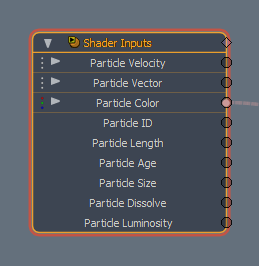Particle Samples

Option

Description

Particle Velocity Output

Outputs the computed Particle Velocity values.

Particle Vector Output

Outputs the computed Particle Vector values.

Particle Color Output

Outputs the computed Particle Color values.

Particle ID Output

Outputs the computed Particle ID value.

Particle Length Output

Outputs the computed length of the particles.

Particle Age Output

Outputs the computed age of the particles since birth.

Particle Size Output

Outputs the computed size of the particles.

Particle Dissolve Output

Outputs the computed transparency of the particles.

Particle Luminosity Output

Outputs the computed luminosity of the particles.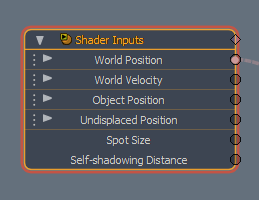Sample Position

Option

Description

World Position Output

Outputs the computed Position of a given sample in world space coordinates.

World Velocity Output

Outputs the computed world velocity of a given sample.

Object Position Output

Outputs the Position of a given sample in Object Space coordinates.

Undisplaced Position Output

Outputs the undisplaced Position of a given sample on a geometric surface in Object Space coordinates.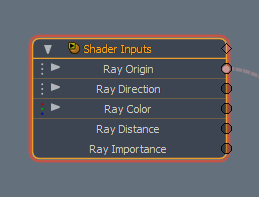Sample Ray

Option

Description

Ray Origin Output

Outputs the computed sample Ray Origin position for a given sample.

Ray Direction Output

Outputs the computed sample Ray Direction for a given sample.

Ray Color Output

Outputs the computed Ray Color for a given sample.

Ray Distance Output

Outputs the computed ray length for a given sample.

Ray Importance Output

Outputs the Rays computed Importance value for a given sample.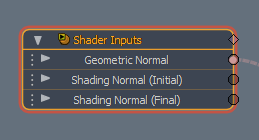Surface Normal

Option

Description

Geometric Normal Output

Outputs the geometric normal of a given sample.

Outputs the unperturbed normal of a given sample.

Outputs the perturbed normal of a given sample.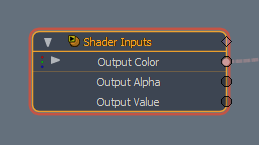Texture Value

Option

Description

Output Color Output

Outputs the computed color of a given sample.

Output Alpha Output

Outputs the computed texture transparency of a given sample.

Output Value Output

Outputs the computed texture value of a given sample.